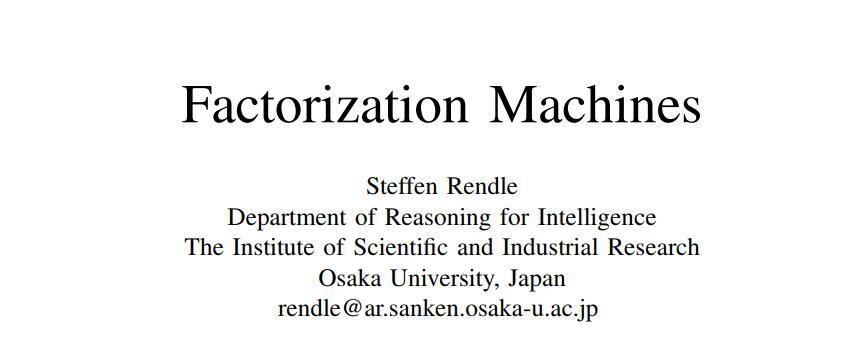## 2. FM原理

FM主要是解决稀疏数据下的特征组合问题，并且其预测的复杂度是线性的，对于连续和离散特征有较好的通用性。## 2.1 特征交叉是两个维向量的内积：## 2.2 预测

FM算法可以应用在多种的预测任务中，包括：

• Regression可以直接用作预测，并且最小平方误差来优化。
• Binary classification作为目标函数并且使用hinge loss或者logit loss来优化。
• Ranking：向量通过的分数排序，并且通过pairwise的分类损失来优化成对的样本## 2.3 参数学习## 2.4 多阶FM

2阶FM可以很容易泛化到高阶：## 2.5 Factorization Machines With FTRL

2.6 总结

FM模型有两个优势：

1. 在高度稀疏的情况下特征之间的交叉仍然能够估计，而且可以泛化到未被观察的交叉
2. 参数的学习和模型的预测的时间复杂度是线性的

FM模型的优化点：

1.特征为全交叉，耗费资源，通常user与user，item与item内部的交叉的作用要小于user与item的交叉

2.使用矩阵计算，而不是for循环计算

3.高阶交叉特征的构造

## 3. FM实践

LLSean/data-mining

movie id | movie title | release date | video release date |
IMDb URL | unknown | Action | Adventure | Animation |
Children's | Comedy | Crime | Documentary | Drama | Fantasy |
Film-Noir | Horror | Musical | Mystery | Romance | Sci-Fi |
Thriller | War | Western |

u.user包括的数据格式为：

user id | age | gender | occupation | zip code

ua.base和ua.test的数据格式为：

user id | item id | rating | timestamp

def onehot_encoder(labels, NUM_CLASSES):
enc = LabelEncoder()
labels = enc.fit_transform(labels)
labels = labels.astype(np.int32)
batch_size = tf.size(labels)
labels = tf.expand_dims(labels, 1)
indices = tf.expand_dims(tf.range(0, batch_size,1), 1)
concated = tf.concat([indices, labels] , 1)
onehot_labels = tf.sparse_to_dense(concated, tf.stack([batch_size, NUM_CLASSES]), 1.0, 0.0)
with tf.Session() as sess:
return sess.run(onehot_labels)

header = ['user_id', 'age', 'gender', 'occupation', 'zip_code']
header = ['item_id', 'title', 'release_date', 'video_release_date', 'IMDb_URL', 'unknown', 'Action', 'Adventure', 'Animation', 'Children',
'Comedy', 'Crime', 'Documentary', 'Drama', 'Fantasy', 'Film-Noir', 'Horror', 'Musical', 'Mystery', 'Romance', 'Sci-Fi',
'Thriller', 'War', 'Western']
df_item = df_item.drop(columns=['title', 'release_date', 'video_release_date', 'IMDb_URL', 'unknown'])

df_user['age'] = pd.cut(df_user['age'], [0,10,20,30,40,50,60,70,80,90,100], labels=['0-10','10-20','20-30','30-40','40-50','50-60','60-70','70-80','80-90','90-100'])
df_user = pd.get_dummies(df_user, columns=['gender', 'occupation', 'age'])
df_user = df_user.drop(columns=['zip_code'])

user_features = df_user.columns.values.tolist()
movie_features = df_item.columns.values.tolist()
cols = user_features + movie_features

header = ['user_id', 'item_id', 'rating', 'timestamp']
df_train['rating'] = df_train.rating.apply(lambda x: 1 if int(x) == 5 else 0)
df_train = df_train.merge(df_user, on='user_id', how='left')
df_train = df_train.merge(df_item, on='item_id', how='left')

df_test['rating'] = df_test.rating.apply(lambda x: 1 if int(x) == 5 else 0)
df_test = df_test.merge(df_user, on='user_id', how='left')
df_test = df_test.merge(df_item, on='item_id', how='left')
train_labels = onehot_encoder(df_train['rating'].astype(np.int32), 2)
test_labels = onehot_encoder(df_test['rating'].astype(np.int32), 2)
return df_train[cols].values, train_labels, df_test[cols].values, test_labels

#输入
self.X = tf.placeholder('float32', [None, self.p])
self.y = tf.placeholder('float32', [None, self.num_classes])
self.keep_prob = tf.placeholder('float32')

#forward过程
def inference(self):
with tf.variable_scope('linear_layer'):
w0 = tf.get_variable('w0', shape=[self.num_classes],
initializer=tf.zeros_initializer())
self.w = tf.get_variable('w', shape=[self.p, num_classes],
initializer=tf.truncated_normal_initializer(mean=0,stddev=0.01))

with tf.variable_scope('interaction_layer'):
self.v = tf.get_variable('v', shape=[self.p, self.k],
initializer=tf.truncated_normal_initializer(mean=0, stddev=0.01))
self.interaction_terms = tf.multiply(0.5,
tf.reduce_sum(
tf.subtract(
tf.pow(tf.matmul(self.X, self.v), 2),
tf.matmul(self.X, tf.pow(self.v, 2))),
1, keep_dims=True))
if self.num_classes == 2:
self.y_out_prob = tf.nn.sigmoid(self.y_out)
elif self.num_classes > 2:
self.y_out_prob = tf.nn.softmax(self.y_out)

#loss
if self.num_classes == 2:
cross_entropy = tf.nn.sigmoid_cross_entropy_with_logits(labels=self.y, logits=self.y_out)
elif self.num_classes > 2:
cross_entropy = tf.nn.softmax_cross_entropy_with_logits(labels=self.y, logits=self.y_out)
mean_loss = tf.reduce_mean(cross_entropy)
self.loss = mean_loss
tf.summary.scalar('loss', self.loss)

#计算accuracy
# accuracy
self.correct_prediction = tf.equal(tf.cast(tf.argmax(self.y_out,1), tf.float32), tf.cast(tf.argmax(self.y,1), tf.float32))
self.accuracy = tf.reduce_mean(tf.cast(self.correct_prediction, tf.float32))
tf.summary.scalar('accuracy', self.accuracy)

#训练
def train(self):
self.global_step = tf.Variable(0, trainable=False)
optimizer = tf.train.FtrlOptimizer(self.lr, l1_regularization_strength=self.reg_l1,
l2_regularization_strength=self.reg_l2)
extra_update_ops = tf.get_collection(tf.GraphKeys.UPDATE_OPS)
with tf.control_dependencies(extra_update_ops):
self.train_op = optimizer.minimize(self.loss, global_step=self.global_step)

#构建图
def build_graph(self):
self.inference()
self.train()

csie.ntu.edu.tw/~b97053